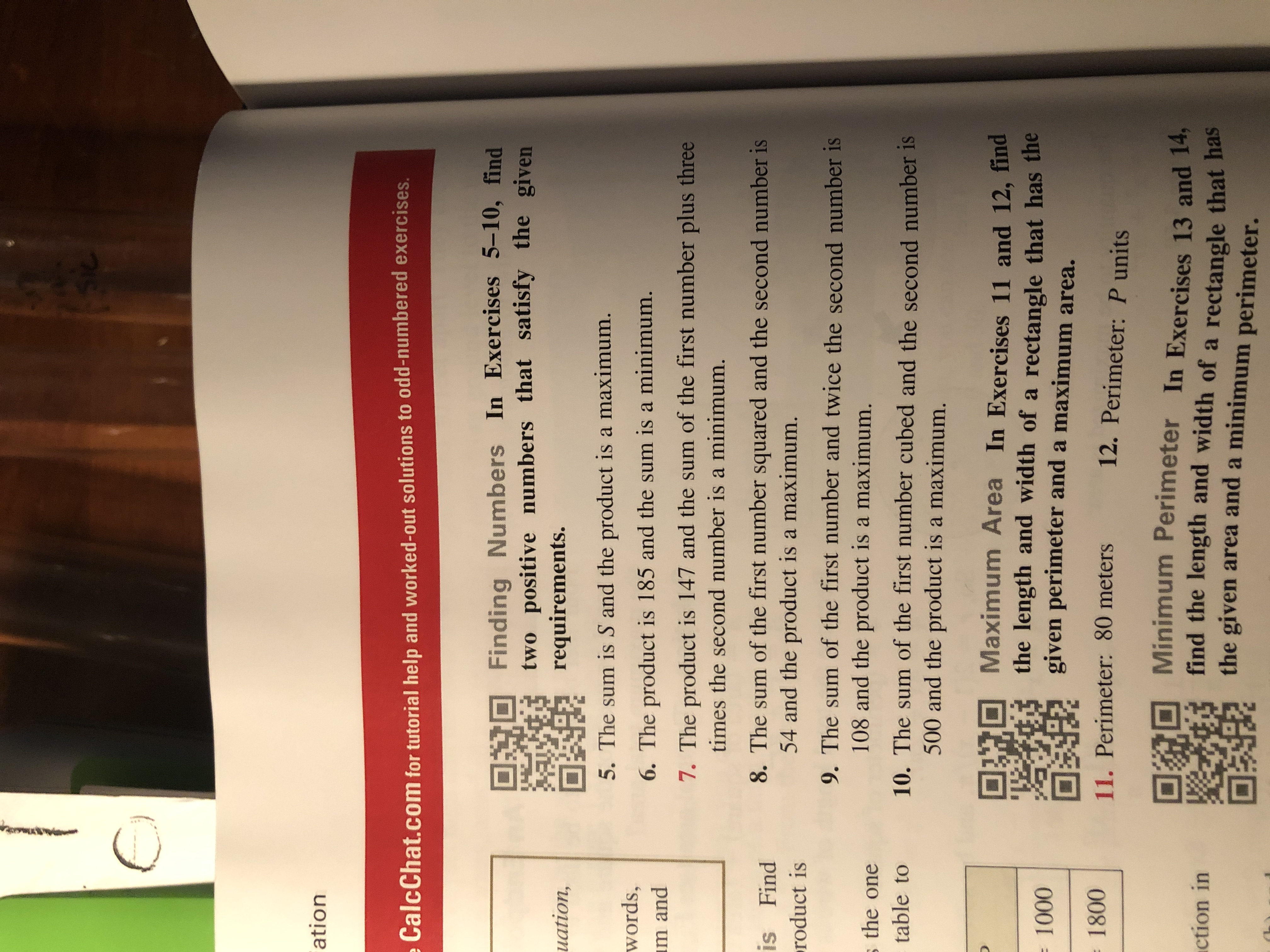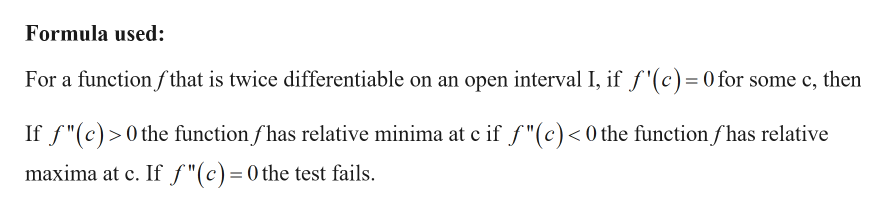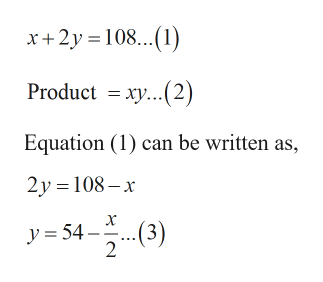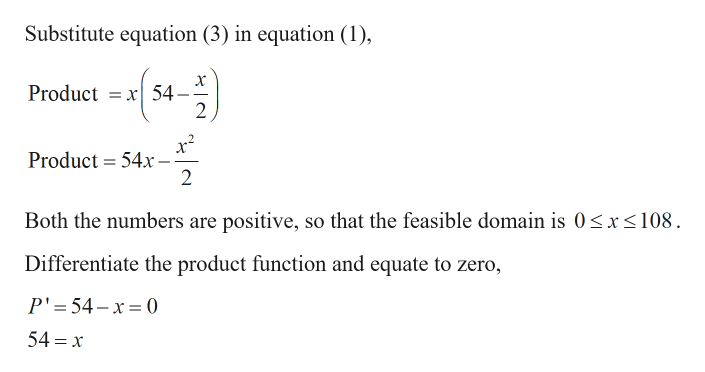# CationCalcChat.com for tutorial help and worked-out solutions to odd-numbered exercisesFinding Numbers In Exercises 5-10, findtwo positive numbers that satisfy the givenrequirements.uation,5. The sum is S and the product is a maximum.words,m and6. The product is 185 and the sum is a minimum.7. The product is 147 and the sum of the first number plus threetimes the second number is a minimum.8. The sum of the first number squared and the second number is54 and the product is a maximum.is Findroduct is9. The sum of the first number and twice the second number is108 and the prOduct is a maximum.the one10. The sum of the first number cubed and the second number is500 and the product is a maximum.table toMaximum Area In Exercises 11 and 12, findthe length and width of a rectangle that has thegiven perimeter and a maximum area.1000180011. Perimeter: 80 meters12. Perimeter: P unitsMinimum Perimeter In Exercises 13 and 14,find the length and width of a rectangle that hasthe given area and a minimum perimeter.ction in

Question
38 views

9help_outlineImage TranscriptioncloseC ation CalcChat.com for tutorial help and worked-out solutions to odd-numbered exercises Finding Numbers In Exercises 5-10, find two positive numbers that satisfy the given requirements. uation, 5. The sum is S and the product is a maximum. words, m and 6. The product is 185 and the sum is a minimum. 7. The product is 147 and the sum of the first number plus three times the second number is a minimum. 8. The sum of the first number squared and the second number is 54 and the product is a maximum. is Find roduct is 9. The sum of the first number and twice the second number is 108 and the prOduct is a maximum. the one 10. The sum of the first number cubed and the second number is 500 and the product is a maximum. table to Maximum Area In Exercises 11 and 12, find the length and width of a rectangle that has the given perimeter and a maximum area. 1000 1800 11. Perimeter: 80 meters 12. Perimeter: P units Minimum Perimeter In Exercises 13 and 14, find the length and width of a rectangle that has the given area and a minimum perimeter. ction in fullscreen
check_circle

Step 1

Given:

The sum of the first number and twice the second number is 108.help_outlineImage TranscriptioncloseFormula used: For a function fthat is twice differentiable on an open interval I, if f'(c)=0 for some c, then If f"(c)0the function fhas relative minima at c if f"(c)<0the function fhas relative maxima at c. If f"(c)=0the test fails fullscreen
Step 2

Calculation:

Let the two numbers be x and y.

From the given information,help_outlineImage Transcriptionclosex+2y 108...) Product xy. 2) Equation (1) can be written as, 2y 108-x (3) y 54 2 fullscreen
Step 3help_outlineImage TranscriptioncloseSubstitute equation (3) in equation (1) - x Product x54 - 2 Product 54x 2 Both the numbers are positive, so that the feasible domain is 0

### Want to see the full answer?

See Solution

#### Want to see this answer and more?

Solutions are written by subject experts who are available 24/7. Questions are typically answered within 1 hour.*

See Solution
*Response times may vary by subject and question.
Tagged in

### Other